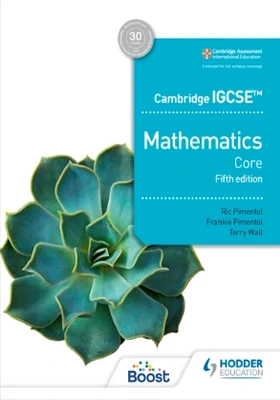Viva Group has been honoured with the Best Publisher Award 2022 by the Delhi State Booksellers & Publishers' Association.

#### Cambridge IGCSE Core Mathematics, 5/e## Cambridge IGCSE Core Mathematics, 5/e

• By: Ric Pimentel, Frankie Pimentel & Terry Wall

₹1,975.50 ₹2,195.00 Save: ₹219.50 (10%)

ISBN: 9781398373938

Bind: Paperback

Year: 2023

Pages: 376

Size: 7.5 x 9.25 Inch

Publisher: Hodder Education

Exclusive Distributors: Viva Books

Sales Territory: India, Nepal, Pakistan, Bangladesh, Sri Lanka

Description:

This title is endorsed by Cambridge Assessment International Education.

Confidently deliver and ensure full coverage of the core content of the latest Cambridge IGCSE™ Mathematics syllabuses (0580/0980) using a tried-and-tested approach to improve mathematical skills with an emphasis on problem-solving and interrogating findings in new topics.

• Put theory into practice with worked examples that show full solutions, plus plenty of exercises, with scaffolding for the appropriate levels, all put together by an experienced team of authors
• Encourage full understanding of mathematical principles with commentaries and additional explanations
• Develop problem-solving skills with guidance on techniques to help complete open-ended investigations and justify reasoning for solutions
• Check understanding with end of chapter student assessment questions to consolidate learning and test skills
• Numerical answers to all questions are available free on hoddereducation.com/cambridgeextras
• Worked solutions for the student assessments are available in the Cambridge IGCSE Core and Extended Mathematics Teacher’s Guide with Boost subscription

Target Audience

IGCSE students studying maths.

Contents

Introduction

TOPIC  1. Number • Chapter 1. Number and language • Chapter 2. Accuracy • Chapter 3. Calculations and order • Chapter 4. Integers, fractions, decimals and percentages • Chapter 5. Further percentages • Chapter 6. Ratio and proportion • Chapter 7. Indices and standard form • Chapter 8. Money and finance • Chapter 9. Time • Chapter 10. Set notation and Venn diagrams • Mathematical investigations and ICT 1

TOPIC  2. Algebra and graphs • Chapter 11. Algebraic representation and manipulation • Chapter 12. Algebraic indices • Chapter 13. Equations • Chapter 14. Sequences • Chapter 15. Graphs in practical situations • Chapter 16. Graphs of functions • Mathematical investigations and ICT 2

TOPIC  3. Coordinate geometry • Chapter 17. Coordinates and straight-line graphs • Mathematical investigations and ICT 3

TOPIC  4. Geometry • Chapter 18. Geometrical vocabulary • Chapter 19. Geometrical constructions and  scale drawings • Chapter 20. Symmetry • Chapter 21. Angle properties • Mathematical investigations and ICT 4

TOPIC  5. Mensuration • Chapter 22. Measures • Chapter 23. Perimeter, area and volume • Mathematical investigations and ICT 5

TOPIC  6. Trigonometry • Chapter 24. Bearings • Chapter 25. Right-angled triangles • Mathematical investigations and ICT 6

TOPIC  7. Vectors and transformations • Chapter 26.  Transformations • Mathematical investigations and ICT 7

TOPIC  8. Probability • Chapter 27. Probability Mathematical investigations and ICT 8

TOPIC  9. Statistics • Chapter 28. Mean, median, mode and range • Chapter 29. Collecting, displaying and interpreting data • Mathematical investigations and ICT 9

Glossary • Index

10%

## Cambridge IGCSE Mathematics Co..

By: Ric Pimentel, Frankie Pimentel..

ISBN : 9781398373914

₹ 1,975.50 ₹ 2,195.00

10%

## Cambridge Primary Mathematics ..

By: Josh Lury and Steph King

ISBN : 9781398301108

₹ 895.50 ₹ 995.00

10%

## Cambridge Primary Mathematics ..

By: Josh Lury and Steph King

ISBN : 9781398301061

₹ 895.50 ₹ 995.00

10%

## Cambridge Primary Mathematics ..

By: Josh Lury and Steph King

ISBN : 9781398301023

₹ 895.50 ₹ 995.00

10%

## Cambridge Primary Mathematics ..

By: Catherine Casey, Josh Lury, St..

ISBN : 9781398300989

₹ 895.50 ₹ 995.00

10%

## Cambridge Primary Mathematics ..

By: Catherine Casey, Josh Lury and..

ISBN : 9781398300941

₹ 895.50 ₹ 995.00